# Solved – How do we define log-normal prior and a multivariate posterior log-likelihood in PyMC

I am a newbie with pyMC and I am not still able to construct the structure of my MCMC with pyMC. I would like to establish a chain and I am confused how to define my parameters and log-likelihood function together. My chi-squared function is given by: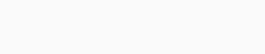whereand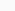are observational data and correspondence error respectively and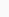is the model with four free parameter and the parameters are non-linear.

The priors for `X` and `Y` are uniform but for `M` and `C` are given as following: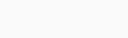;

where the probability of `c` follows log-normal distribution while the expectation value of `c` is computed with the above formula and is the function of `M` and \$sigma\$ is `0.09` if \$M < 10^{15}\$ otherwise \$sigma=0.06\$: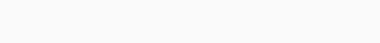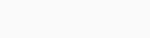for each `C` the parameter `z` is constant. I am wondering how I could define my likelihood for, and should it be referred as @Deterministic variable? Did I define `M` and `C` as priori information in a correct way or not?
I will be grateful if somebody gives me some tips that how I can combine these parameters with given priors.

``import pymc as pm import numpy as np import math import random from scipy.stats import expon  @pm.stochastic(dtype=np.float, observed=False, trace=True) def Xpos(value=1900,x_l=1800,x_h=1950):     """The probable region of the position of halo centre"""     def logp(value,x_l,x_h):         if ((value>x_h) or (value<x_l)):        return -np.inf     else:        return -np.log(x_h-x_l+1)     def random(x_l,x_h):         return np.round((x_h-x_l)*random.random())+x_l  @pm.stochastic(dtype=np.float, observed=False, trace=True) def Ypos(value=1750,y_l=1200,y_h=2000):     """The probable region of the position of halo centre"""     def logp(value,y_l,y_h):         if ((value>y_h) or (value<y_l)):        return -np.inf     else:        return -np.log(y_h-y_l+1)     def random(y_l,y_h):         return np.round((y_h-y_l)*random.random())+y_l   M=math.pow(10,15)*pm.Exponential('mass', beta=math.pow(10,15))  @pm.stochastic(dtype=np.float, observed=False, trace=True) def concentration(value=4, zh, M200): #c parameter     """logp for concentration parameter"""     def logp(value=4.,zh, M):         if (value>0):        x = np.linspace(math.pow(10,13),math.pow(10,16),200 )        prob=expon.pdf(x,loc=0,scale=math.pow(10,15))        conc = [5.26/(1.+zh)*math.pow(x[i]/math.pow(10,14),-0.1) for i in range(len(x))]        mu_c=0        for i in range(len(x)):            mu_c+=prob[i]*conc[i]/sum(prob)        if (M < pow(10,15)):           tau=1./(0.09*0.09)        else:           tau=1./(0.06*0.06)            return  pm.lognormal_like(value, mu_c, tau)         else            return -np.inf     def random(mu_c,tau):         return np.random.lognormal(mu_c, tau, 1) ``
Contents

Your @stochastic uses are not correct. Notice that your functions don't return anything. When using the decorator, you're supposed to return value of the logp. See here for example usage.

If you're going to use @stochastic I think you probably want something like this for each of your @stochastic uses.

``@pm.stochastic(dtype=np.float, observed=False, trace=True) def Xpos(value=1900,x_l=1800,x_h=1950):     """The probable region of the position of halo centre"""      if ((value>x_h) or (value<x_l)):        return -np.inf     else:        return -np.log(x_h-x_l+1) ``

(directly returning the logp)

If you need to provide a random() function (I suspect you don't), I think you can pass it to stochastic.

However, since you just want a uniform prior for Xpos and Ypos, you can just use `Uniform` instead.

``Xpos = Uniform("Xpos", 1800, 1950) ``

Your concentration stochastic seems very complicated given that C is pretty straightforward. I would expect it to directly follow your definition of C.

g_hat should definitely be a @deterministic, since you say its a function. If so, it shouldn't have a likelihood of its own.

For concentration, you want something like

``@deterministic def sigma(value = 1, M=M):     if M > 10**15:        return .09    else:        return .06  cExpected = const/(1+z)*M**-.1 concentration = Lognormal("concentration", cExpected, sigma) ``

Rate this post